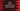# Dart program to convert degree to radian and vice versa## Dart program to convert degree to radian and vice versa:

This program will show you how to convert a value in degree to radian and vice versa. 360° is equal to radians. So, is equal to 2π/360 radian or 0.0174533 radian. Similarly, 1 radian is equal to 360/2π degree.

For raidan, degree conversion, we can write our own methods or we can use a third party library to do that. In this post, I will show you both of these ways.

### Write own function to convert degree to radian and vice versa:

Let’s take a look at the below program:

``````double PI = 3.141592653589793238;

return degree * PI / 180;
}

return radian * 180 / PI;
}

void main() {
List<double> radianValues = [0, 1, 5, 10, 20];
List<double> degreeValues = [0, 57.29577951308232, 90, 360];

}

for (double degree in degreeValues) {
}
}``````

Here,

• PI is a double variable to hold the value of π.
• degreeToRadian method is used to convert one degree value to radian. It returns the result in double.
• radianValues is a list of values in radian we wants to convert to degree. Similarly, degreeValues is a list of values in degree that we will convert to radian.
• Using two for-in loops, we are converting all radian values to degree and all degree values to radian. The print statements are used to print these values to the user.

### Output:

This program will print the below output:

``````0.0 Radian = 0.0 Degree

## Using vector_math library:

vector_math library provides different properties and methods for degree to radian conversion. This is a third party library. Use the below in your pubspec.yaml file:

``````dependencies:
vector_math: any``````

Run pub get to install the library.

Use the below import to import the library:

``import 'package:vector_math/vector_math.dart';``

We can use the below constants:

``degrees2Radians → const double``

It converts an angle from degree to radian. And:

``radians2Degrees → const double``

It gives degree value from radian.

We can also use the below method:

``degrees(double radians) → double``

and:

``radians(double degrees) → double``

Both of these methods takes one double value and returns the required double value.